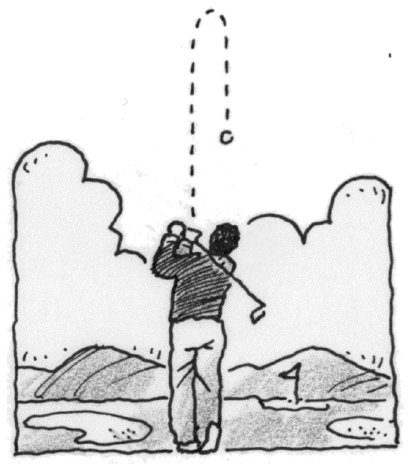### Home > APCALC > Chapter 5 > Lesson 5.2.4 > Problem5-95

5-95.

When Regit hit his golf ball at the $18$th hole, it went straight up in the air!

1. If he hit it with an initial velocity of $144$ feet per second, write an equation for the ball’s velocity, $v\left(t\right)$, at time $t$. Assume the gravitational constant $a\left(t\right) = –32$ ft/sec2 and that Regit hit the ball while it was on the ground.

$s(t)=\frac{1}{2}at^{2}+v_{0}t+s_{0}$

Find $s\left(t\right)$. Then find $v\left(t\right)$.

$s\left(t\right)=−16t^2+144t+0$
$v\left(t\right) = −32t + 144$

2. When is the velocity of the ball $0$ ft/sec? Describe the ball’s position at that moment.

The ball is at rest whenever the velocity is equal to zero. What is happening to the ball at these times?

3. What was the maximum height of the ball?

Remember that the ball has a velocity of zero at the peak of its parabolic flight.